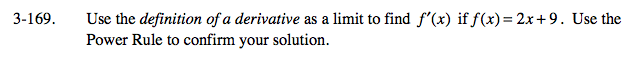### Home > CALC > Chapter 3 > Lesson 3.4.2 > Problem3-169

3-169.

Use the definition of a derivative as a limit to find f ′(x) if f(x) = 2x + 9. Use the Power Rule to confirm your solution. Homework Help ✎Hana's definition of the derivative:

Substitute f(x) into Hana's definition of the derivative.

Expand the numerator and combine like terms.

Factor out an h from the numerator so you can 'cancel out' the h from the denominator.

Evaluate the limit as h →0. This will give you f '(x).

Confirm that the f '(x) you found Algebraically agrees with what you get using the Power Rule.